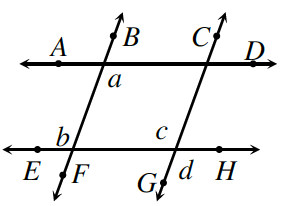Home > CCG > Chapter Ch7 > Lesson 7.2.6 > Problem7-113

7-113.

Penn started the proof below to show that if $\overline{AD}//\overline{EH}$ and $\overline{BF}//\overline{CG}$, then $a=d$. Unfortunately, he did not provide reasons for his proof. Copy his proof and provide a justification for each statement.

For each numbered statement, write how you know it to be true. Think about how angles and parallel lines relate to each other.

Statements

Reasons

1. $\overline{AD}//\overline{EH}$ and $\overline{BF}//\overline{CG}$

Given information.

1. $a=b$

When lines are parallel, then the alternate interior angles are congruent.

1. $b=c$

1. $a=c$

1. $c=d$

1. $a=d$